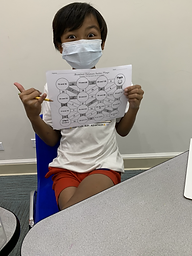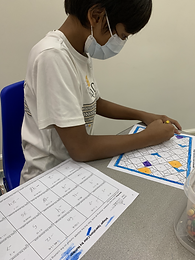## Ms. Kayla

### Target 1​

###### Lesson Type:

Review

Number Operation

:

Integer Composition

Understand that a number can be decomposed into its factors.

###### 1:

Understand factor pairs as two whole numbers that multiple together to get one product.

###### 2:

Find all the factor pairs of a number.

###### 3:

Understand that different numbers can share factors.

5th

###### Vocabulary:

Factor, Multiple, Greatest Common Factor (GCF)

Activities:

1. Students completed a maze by finding the greatest common factor (GCF).

2. Students were given two multiples and listed their factor pairs to find the greatest common factor.### Home Exploration

###### Guiding Questions:## Absent Students:

### Target 2

:

###### 1:

Understand that numbers have an unchanging order, so even if a number is not written on the number line (it still has a space).

###### 2:

Indicate an integer on the number line.

6th

###### Vocabulary:

Positive, Negative, Integer, Number line

Activities:

1. Students used a number line to solve mathematical expressions involving positive and negative integers. The expressions included addition or subtraction of positive and/or negative integers.### Home Exploration

###### Guiding Questions:### Target 3

:

###### Vocabulary:

Activities:### Home Exploration